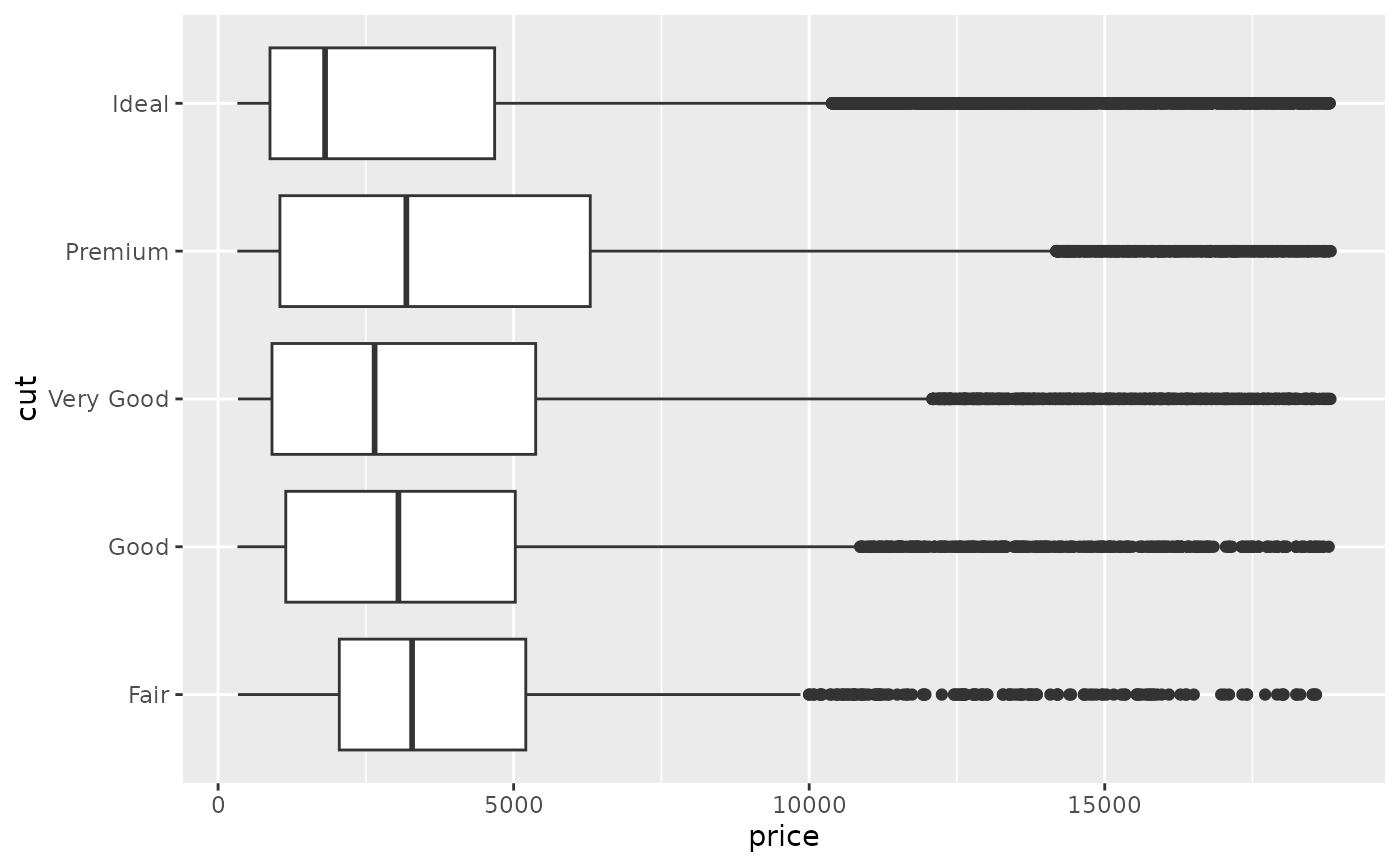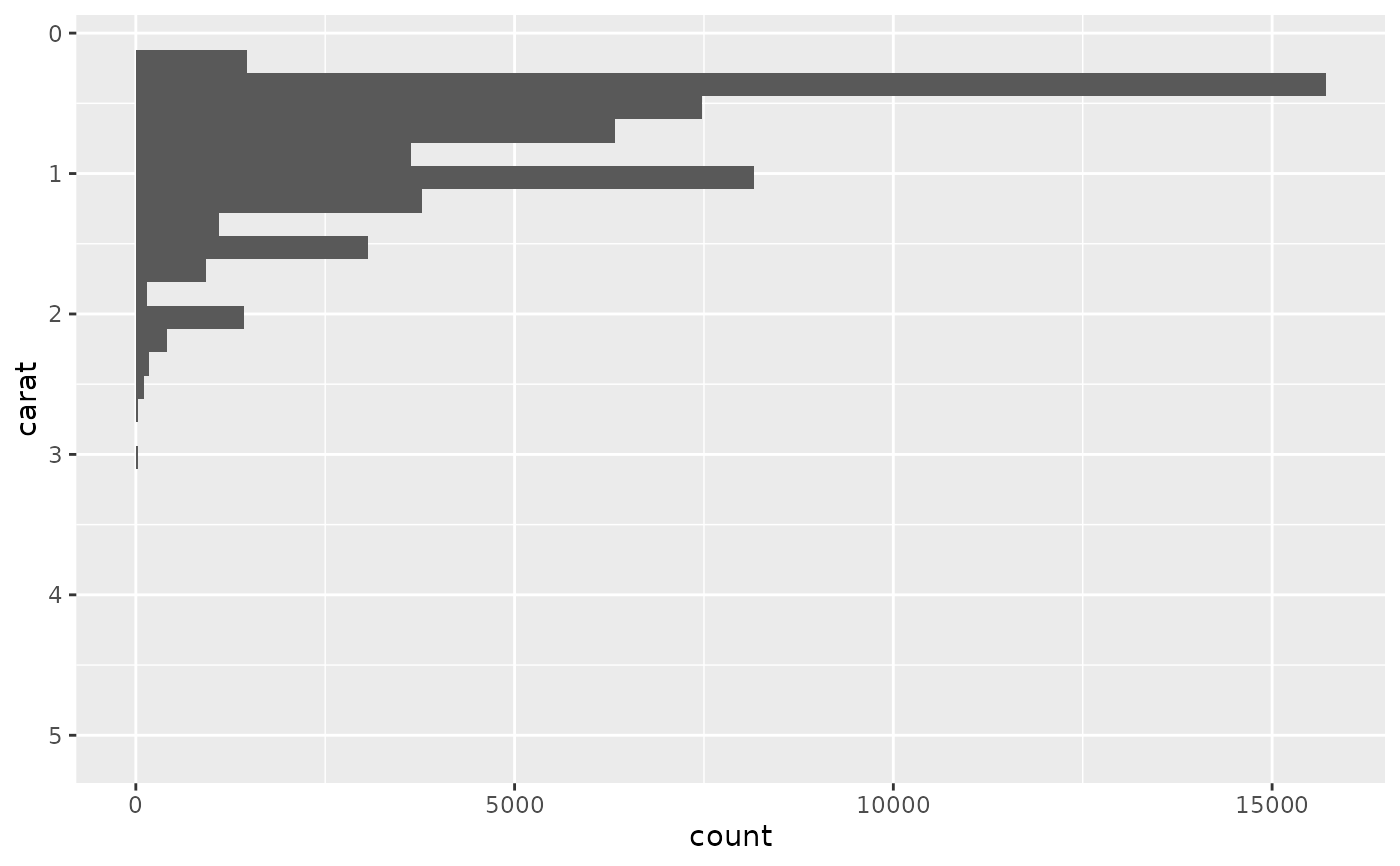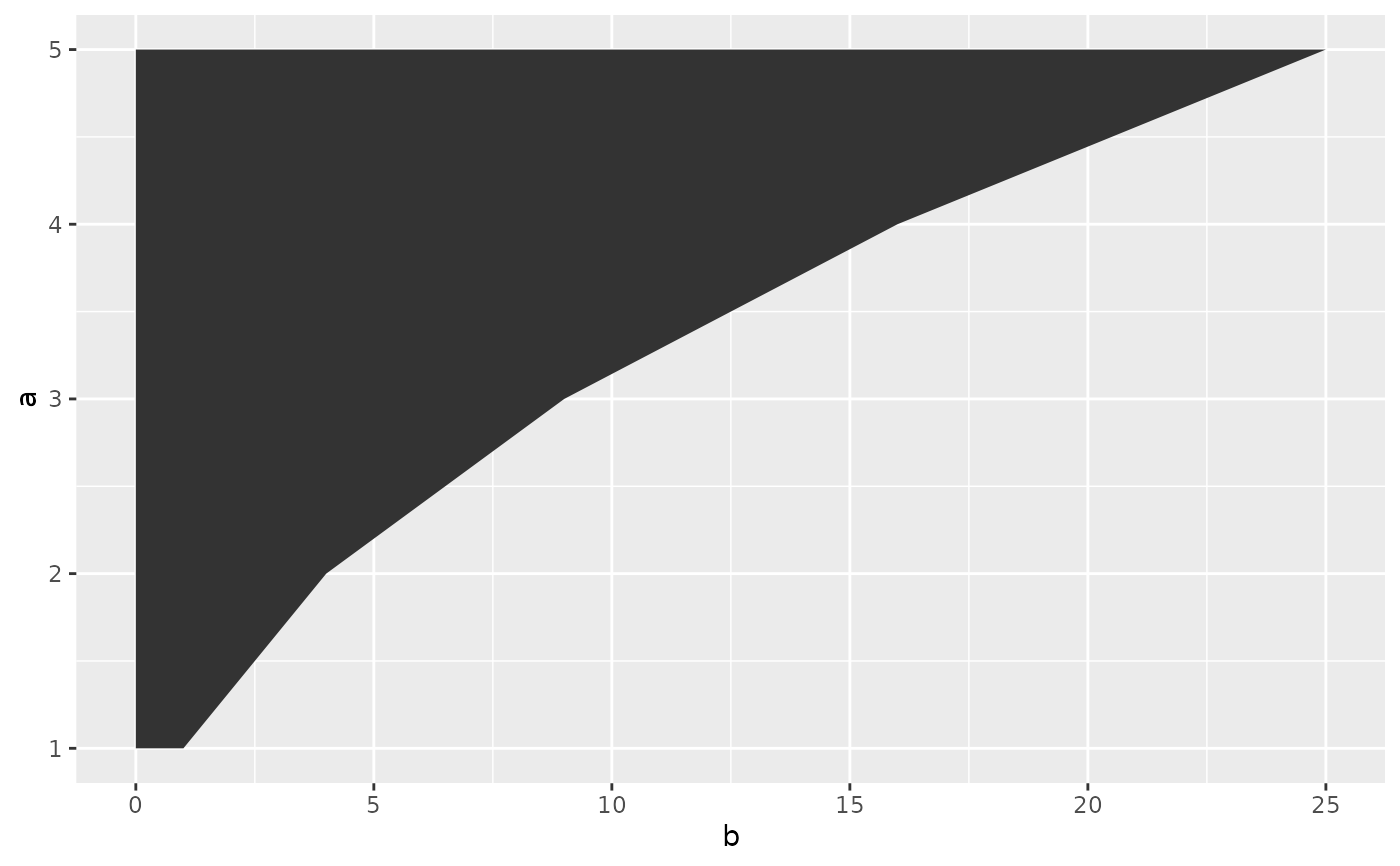Flip cartesian coordinates so that horizontal becomes vertical, and vertical, horizontal. This is primarily useful for converting geoms and statistics which display y conditional on x, to x conditional on y.

## Usage

coord_flip(xlim = NULL, ylim = NULL, expand = TRUE, clip = "on")

## Arguments

xlim, ylim

Limits for the x and y axes.

expand

If TRUE, the default, adds a small expansion factor to the limits to ensure that data and axes don't overlap. If FALSE, limits are taken exactly from the data or xlim/ylim.

clip

Should drawing be clipped to the extent of the plot panel? A setting of "on" (the default) means yes, and a setting of "off" means no. In most cases, the default of "on" should not be changed, as setting clip = "off" can cause unexpected results. It allows drawing of data points anywhere on the plot, including in the plot margins. If limits are set via xlim and ylim and some data points fall outside those limits, then those data points may show up in places such as the axes, the legend, the plot title, or the plot margins.

## Examples

# Very useful for creating boxplots, and other interval
# geoms in the horizontal instead of vertical position.

ggplot(diamonds, aes(cut, price)) +
geom_boxplot() +
coord_flip()h <- ggplot(diamonds, aes(carat)) +
geom_histogram()
h
#> stat_bin() using bins = 30. Pick better value with binwidth.h + coord_flip()
#> stat_bin() using bins = 30. Pick better value with binwidth.h + coord_flip() + scale_x_reverse()
#> stat_bin() using bins = 30. Pick better value with binwidth.# You can also use it to flip line and area plots:
df <- data.frame(x = 1:5, y = (1:5) ^ 2)
ggplot(df, aes(x, y)) +
geom_area()last_plot() + coord_flip()